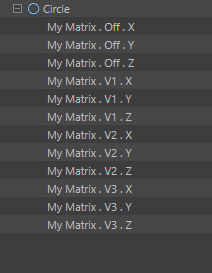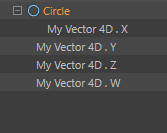# Keyframing UserData with Value Tracks

• Hello,
I'm trying to set keyframes for any type of UserData that can be set with a float value (Value CTracks). I believe those are Real, Long, Vector, Vector 4D, Color, & Matrix. Using this article from Cineversity as my guide, I've hit three issues and could use some help.

1. Setting the Min & Max values for Vectors
I want to get the min & max values for a CTrack so if I try to set the value to something out of range, it defaults to either the minimum or maximum value. I am confused how to go about this.

2. Keyframing the 'x', 'y', & 'z' of the User Data's Matrix Vectors
Currently I'm just setting keyframes on the Matrix Vectors themselves, not their 'x', 'y', & 'z' values. I can tell because the CTracks do not belong to the object and when I keyframe manually, they do.
This is how it should look (this was done with manual keyframing):3. Getting 'w' CTrack in Vector 4D Data Type
When keyframed manually, Vector 4D shows a CTrack for 'w' but there doesn't seem to be a `VECTOR_W`.
This is with manual keyframing:My results (from the code below) do not target the Matrix's x, y, z and neither those tracks nor the Vector 4D are not being added to the Circle's User Data group:Here is my code and a scene file with an object that has many userdata types:

``````import c4d

def GetUDCtracks(op,id):
cTracks = []
ud = id
dtype = ud.dtype
if dtype in (c4d.DTYPE_VECTOR,c4d.DTYPE_COLOR):
for v in xrange(c4d.VECTOR_X, c4d.VECTOR_Z+1):
descID = c4d.DescID(id,id,c4d.DescLevel(v,c4d.DTYPE_REAL))
cTracks.append(c4d.CTrack(op,descID))
elif dtype == c4d.DTYPE_VECTOR4D:
for v in xrange(c4d.VECTOR_X, c4d.VECTOR_Z+2): # How to get Vector4D.w?
# this is not adding under the correct user data group
descID = c4d.DescID(id,id,c4d.DescLevel(v,c4d.DTYPE_REAL))
cTracks.append(c4d.CTrack(op,descID))
elif dtype == c4d.DTYPE_MATRIX:
# This is not adding the correct cTrack (x,y, or z) to the cTracks list
# nor is it adding under the correct user data group
for v in xrange(c4d.MATRIX_OFF, c4d.MATRIX_V3+1):
descID = c4d.DescID(id,id,c4d.DescLevel(v,c4d.DTYPE_LONG))
for i in xrange(c4d.VECTOR_X, c4d.VECTOR_Z+2):
descID2 = c4d.DescID(descID,descID,c4d.DescLevel(i,c4d.DTYPE_REAL))
cTracks.append(c4d.CTrack(op,descID2))
else:
cTracks.append(c4d.CTrack(op,id))
return cTracks

def main(doc):
doc.StartUndo()
value = 1.0
keyTime = doc.GetTime()
for id, bc in op.GetUserDataContainer():
dtype = id.dtype
print "dtype: %s"%dtype
print "bc[c4d.DESC_NAME]: %s"%bc[c4d.DESC_NAME]

if dtype not in (c4d.DTYPE_MATRIX,c4d.DTYPE_REAL,c4d.DTYPE_VECTOR,
c4d.DTYPE_VECTOR4D, c4d.DTYPE_LONG, c4d.DTYPE_COLOR):
continue

print "bc[c4d.DESC_MAX]: %s"%bc[c4d.DESC_MAX]
print "bc[c4d.DESC_MIN]: %s"%bc[c4d.DESC_MIN]

cTrack = op.FindCTrack(id)
if not cTrack:
cTracks = GetUDCtracks(op,id)
for track in cTracks:
print track
op.InsertTrackSorted(track)
curve = track.GetCurve()
myKey = keyDict["key"]
track.FillKey(doc,op,myKey)

# How can I check the curve's min & max before setting the value
# if it's one dimension of a Vector?

myKey.SetValue(curve,value)

doc.EndUndo()

if __name__=='__main__':
main(doc)
``````

Keyframing UserData.c4d

Any help with this would be tremendous! Thank you• The main issue is that in your GetUDCtracks you always create a new track and search within this track, so obviously there is no key already presents.

``````import c4d

def CopyDescID(descIDToCopy):
buffer = c4d.DescID()
for x in xrange(descIDToCopy.GetDepth()):
buffer.PushId(descIDToCopy[x])

return buffer

def GetAllChildrenDescId(baseDescId):
results = []

# Hardcode in a dict, the Type and a list of ID sub entry
tracksData = {}
tracksData[c4d.DTYPE_VECTOR] = [c4d.DTYPE_REAL, [c4d.VECTOR_X, c4d.VECTOR_Y, c4d.VECTOR_Z]]
tracksData[c4d.DTYPE_COLOR] = [c4d.DTYPE_REAL, [c4d.COLOR_R, c4d.COLOR_G, c4d.COLOR_B]]
tracksData[c4d.DTYPE_VECTOR4D] = [c4d.DTYPE_REAL, [c4d.VECTOR4D_X, c4d.VECTOR4D_Y, c4d.VECTOR4D_Z, c4d.VECTOR4D_W]]
tracksData[c4d.DTYPE_MATRIX] = [c4d.DTYPE_VECTOR, [c4d.MATRIX_OFF, c4d.MATRIX_V1, c4d.MATRIX_V2, c4d.MATRIX_V3]]

# Retrieve the last part of the descId and check if it in our tracksData dict, otherwise return
dtype = baseDescId[-1].dtype
descIdData = tracksData.get(dtype)
if descIdData is None:
return [baseDescId]

# For each entry of the Base
for descId in descIdData:
# Get a new copy of the baseDescID and add the current level
descLevel = c4d.DescLevel(descId, descIdData, 0)
newDescId = CopyDescID(baseDescId)
newDescId.PushId(descLevel)

'''
Recursivly checks all descId (eg a matrix will generate vector,
so we need then to process each vector)
'''
resolvedDescIds = GetAllChildrenDescId(newDescId)

# If there is some results we add the result to the final list
if len(resolvedDescIds) > 1:
for resolvedDescId in resolvedDescIds:
results.append(resolvedDescId)

# Otherwise we create one
else:
results.append(newDescId)

return results

def GetUDCtracks(op, baseDescId):
cTracks = []
allDescId = GetAllChildrenDescId(baseDescId)

for descId in allDescId:
track = op.FindCTrack(descId)
if track is None:
track = c4d.CTrack(op, descId)
op.InsertTrackSorted(track)

cTracks.append(track)

return cTracks

def main(doc):
doc.StartUndo()
value = 1.0
keyTime = doc.GetTime()
fps = doc.GetFps()
frame = keyTime.GetFrame(doc.GetFps())

for descID, bc in op.GetUserDataContainer():
dtype = descID.dtype

if dtype not in (c4d.DTYPE_MATRIX,c4d.DTYPE_REAL,c4d.DTYPE_VECTOR,
c4d.DTYPE_VECTOR4D, c4d.DTYPE_LONG, c4d.DTYPE_COLOR):
continue

# This will retrieve or create the track if it doesnt exist
cTracks = GetUDCtracks(op, descID)
for cTrack in cTracks:
curve = cTrack.GetCurve()

keyDict = curve.FindKey(keyTime)
# Never checks None by == operator but always use is
if keyDict is None:
key = keyDict["key"]

if keyDict is None:
raise RuntimeError('key is None, this should never happens')

key = keyDict["key"]
key.SetValue(curve,value)

doc.EndUndo()

if __name__=='__main__':
main(doc)
``````

Cheers,
Maxime.

• Hi @blastframe,

1. There is no way to define a minimal and a maximal value in a Curve, the only way would be to edit each Ckey and define their values.
However keep in mind this is the parameter that wins at the end, so if I have a float parameter limited to 1.0.
I can define animation going from 0 to 2000.0 nothing prevents me, but when Cinema 4D will evaluate the Animation track, it will then clamp the value according to the parameter max value so 1.0.

2. You have to hardcode everything, here its one way:

``````def CopyDescID(descIDToCopy):
buffer = c4d.DescID()
for x in xrange(descIDToCopy.GetDepth()):
buffer.PushId(descIDToCopy[x])

return buffer

def GetAllChildrenDescId(baseDescId):
results = []

# Hardcode in a dict, the Type and a list of ID sub entry
tracksData = {}
tracksData[c4d.DTYPE_VECTOR] = [c4d.DTYPE_REAL, [c4d.VECTOR_X, c4d.VECTOR_Y, c4d.VECTOR_Z]]
tracksData[c4d.DTYPE_COLOR] = [c4d.DTYPE_REAL, [c4d.COLOR_R, c4d.COLOR_G, c4d.COLOR_B]]
tracksData[c4d.DTYPE_VECTOR4D] = [c4d.DTYPE_REAL, [c4d.VECTOR4D_X, c4d.VECTOR4D_Y, c4d.VECTOR4D_Z, c4d.VECTOR4D_W]]
tracksData[c4d.DTYPE_MATRIX] = [c4d.DTYPE_VECTOR, [c4d.MATRIX_OFF, c4d.MATRIX_V1, c4d.MATRIX_V2, c4d.MATRIX_V3]]

# Retrieve the last part of the descId and check if it in our tracksData dict, otherwise return
dtype = baseDescId[-1].dtype
descIdData = tracksData.get(dtype)
if descIdData is None:
return [baseDescId]

# For each entry of the Base
for descId in descIdData:
# Get a new copy of the baseDescID and add the current leve
descLevel = c4d.DescLevel(descId, descIdData, 0)
newDescId = CopyDescID(baseDescId)
newDescId.PushId(descLevel)

# Recursivly checks all descId (eg a matrix will generate vector, so we need then to process each vector)
resolvedDescIds = GetAllChildrenDescId(newDescId)

# If there is some results we had the result to the final list
if len(resolvedDescIds) > 1:
for resolvedDescId in resolvedDescIds:
results.append(resolvedDescId)

# Otherwise we the created one
else:
results.append(newDescId)

return results

def GetUDCtracks(op, baseDescId):
cTracks = []
allDescId = GetAllChildrenDescId(baseDescId)

for descId in allDescId:
cTracks.append(c4d.CTrack(op, descId))

return cTracks
``````
1. It's called `VECTOR4D_W`.

Cheers,
Maxime

• @m_adam Hi Maxime, thank you for the reply. Your post was very helpful but I'm still have an issue: the FindKey, AddKey, GetKeyCount methods aren't working properly for me with this code. If I move my playhead in the timeline, I'm able to get the track, but unable to create more than one keyframe. My script cannot see any of the keyframes in the curve. Maybe I am missing something simple. Here's the combined script:

``````import c4d

def CopyDescID(descIDToCopy):
buffer = c4d.DescID()
for x in xrange(descIDToCopy.GetDepth()):
buffer.PushId(descIDToCopy[x])

return buffer

def GetAllChildrenDescId(baseDescId):
results = []

# Hardcode in a dict, the Type and a list of ID sub entry
tracksData = {}
tracksData[c4d.DTYPE_VECTOR] = [c4d.DTYPE_REAL, [c4d.VECTOR_X, c4d.VECTOR_Y, c4d.VECTOR_Z]]
tracksData[c4d.DTYPE_COLOR] = [c4d.DTYPE_REAL, [c4d.COLOR_R, c4d.COLOR_G, c4d.COLOR_B]]
tracksData[c4d.DTYPE_VECTOR4D] = [c4d.DTYPE_REAL, [c4d.VECTOR4D_X, c4d.VECTOR4D_Y, c4d.VECTOR4D_Z, c4d.VECTOR4D_W]]
tracksData[c4d.DTYPE_MATRIX] = [c4d.DTYPE_VECTOR, [c4d.MATRIX_OFF, c4d.MATRIX_V1, c4d.MATRIX_V2, c4d.MATRIX_V3]]

# Retrieve the last part of the descId and check if it in our tracksData dict, otherwise return
dtype = baseDescId[-1].dtype
descIdData = tracksData.get(dtype)
if descIdData is None:
return [baseDescId]

# For each entry of the Base
for descId in descIdData:
# Get a new copy of the baseDescID and add the current level
descLevel = c4d.DescLevel(descId, descIdData, 0)
newDescId = CopyDescID(baseDescId)
newDescId.PushId(descLevel)

'''
Recursivly checks all descId (eg a matrix will generate vector,
so we need then to process each vector)
'''
resolvedDescIds = GetAllChildrenDescId(newDescId)

# If there is some results we add the result to the final list
if len(resolvedDescIds) > 1:
for resolvedDescId in resolvedDescIds:
results.append(resolvedDescId)

# Otherwise we create one
else:
results.append(newDescId)

return results

def GetUDCtracks(op, baseDescId):
cTracks = []
allDescId = GetAllChildrenDescId(baseDescId)

for descId in allDescId:
cTracks.append(c4d.CTrack(op, descId))

return cTracks

def main(doc):
doc.StartUndo()
value = 1.0
keyTime = doc.GetTime()
fps = doc.GetFps()
frame = keyTime.GetFrame(doc.GetFps())

for id, bc in op.GetUserDataContainer():
dtype = id.dtype

if dtype not in (c4d.DTYPE_MATRIX,c4d.DTYPE_REAL,c4d.DTYPE_VECTOR,
c4d.DTYPE_VECTOR4D, c4d.DTYPE_LONG, c4d.DTYPE_COLOR):
continue

cTrack = op.FindCTrack(id)
if not cTrack:
cTracks = GetUDCtracks(op,id)
for track in cTracks:
op.InsertTrackSorted(track)
curve = track.GetCurve()
key = keyDict["key"]
key.SetValue(curve,value)
keyCount = curve.GetKeyCount()
print "frame: %s, track: %s, keyCount: %s"\
%(frame,track.GetName(),keyCount)
else:
cTracks = GetUDCtracks(op,id)
for track in cTracks:
curve = track.GetCurve()
findKey = curve.FindKey(keyTime);
keyCount = curve.GetKeyCount()
if findKey == None:
print "frame: %s, track: %s, keyCount: %s, "\
"findKey: %s"%(frame,track.GetName(),
keyCount,findKey)
key = keyDict["key"]
key.SetValue(curve,value)

doc.EndUndo()

if __name__=='__main__':
main(doc)
``````

Is there a special method for User Data CTracks?

• The main issue is that in your GetUDCtracks you always create a new track and search within this track, so obviously there is no key already presents.

``````import c4d

def CopyDescID(descIDToCopy):
buffer = c4d.DescID()
for x in xrange(descIDToCopy.GetDepth()):
buffer.PushId(descIDToCopy[x])

return buffer

def GetAllChildrenDescId(baseDescId):
results = []

# Hardcode in a dict, the Type and a list of ID sub entry
tracksData = {}
tracksData[c4d.DTYPE_VECTOR] = [c4d.DTYPE_REAL, [c4d.VECTOR_X, c4d.VECTOR_Y, c4d.VECTOR_Z]]
tracksData[c4d.DTYPE_COLOR] = [c4d.DTYPE_REAL, [c4d.COLOR_R, c4d.COLOR_G, c4d.COLOR_B]]
tracksData[c4d.DTYPE_VECTOR4D] = [c4d.DTYPE_REAL, [c4d.VECTOR4D_X, c4d.VECTOR4D_Y, c4d.VECTOR4D_Z, c4d.VECTOR4D_W]]
tracksData[c4d.DTYPE_MATRIX] = [c4d.DTYPE_VECTOR, [c4d.MATRIX_OFF, c4d.MATRIX_V1, c4d.MATRIX_V2, c4d.MATRIX_V3]]

# Retrieve the last part of the descId and check if it in our tracksData dict, otherwise return
dtype = baseDescId[-1].dtype
descIdData = tracksData.get(dtype)
if descIdData is None:
return [baseDescId]

# For each entry of the Base
for descId in descIdData:
# Get a new copy of the baseDescID and add the current level
descLevel = c4d.DescLevel(descId, descIdData, 0)
newDescId = CopyDescID(baseDescId)
newDescId.PushId(descLevel)

'''
Recursivly checks all descId (eg a matrix will generate vector,
so we need then to process each vector)
'''
resolvedDescIds = GetAllChildrenDescId(newDescId)

# If there is some results we add the result to the final list
if len(resolvedDescIds) > 1:
for resolvedDescId in resolvedDescIds:
results.append(resolvedDescId)

# Otherwise we create one
else:
results.append(newDescId)

return results

def GetUDCtracks(op, baseDescId):
cTracks = []
allDescId = GetAllChildrenDescId(baseDescId)

for descId in allDescId:
track = op.FindCTrack(descId)
if track is None:
track = c4d.CTrack(op, descId)
op.InsertTrackSorted(track)

cTracks.append(track)

return cTracks

def main(doc):
doc.StartUndo()
value = 1.0
keyTime = doc.GetTime()
fps = doc.GetFps()
frame = keyTime.GetFrame(doc.GetFps())

for descID, bc in op.GetUserDataContainer():
dtype = descID.dtype

if dtype not in (c4d.DTYPE_MATRIX,c4d.DTYPE_REAL,c4d.DTYPE_VECTOR,
c4d.DTYPE_VECTOR4D, c4d.DTYPE_LONG, c4d.DTYPE_COLOR):
continue

# This will retrieve or create the track if it doesnt exist
cTracks = GetUDCtracks(op, descID)
for cTrack in cTracks:
curve = cTrack.GetCurve()

keyDict = curve.FindKey(keyTime)
# Never checks None by == operator but always use is
if keyDict is None:
key = keyDict["key"]

if keyDict is None:
raise RuntimeError('key is None, this should never happens')

key = keyDict["key"]
key.SetValue(curve,value)

• @m_adam You are a genius! Thank you, Maxime